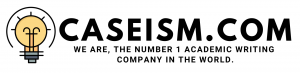# Fixed and Floating rate Case Solution & Answer

## Â Fixed and Floating rate Case Solution

Fixed rate payments: In the case of fixed rate swap, the rate would remain same that is 2.6%, and it pays the total amount of 1.3 million semi-annually. The LIBOR is used as by the recent statistic of the last three years as shown in below table and 0.5% is added as it keeps on changing with the fixed rate.

 1 16-Jan 0.6117% 2 Apr-16 0.6291% 3 Sep-15 0.3340%

Here we are using Fixed to Floating SWAP rate, which shows the investor receives the fixed amount of about 1.3 million and pays 1.11 million, 1.129 million and 0.834 million in respective years. The net cash flow results in \$ 188,300, \$170,900 and \$ 466,000 in year 1, 2 and 3 and this resulted in positive cash flow of \$ 825,200. This investment would be suggested as profitable.

Floating rate payment:

The floating rate payment is made on three months LIBOR and the ask rate of 1.32%. It is calculated as 1.32% plus the three months recent LIBOR as shown in above table. Therefore, this resulted in different rates such as 1.93%, 1.95% and 1.65% in three years.

Over here we use floating to fixed rates, in this swap the investor has to pay fixed amount and receive the floating amount. The investor receives the different amount based on the floating rates as it keeps on changing, which more than fixed amount is paid. The investor receives the net cash flow after differentiating the results of about \$ 1.634 million, which is more profitable as compared to the fixed rate swap.

The value of Net cash flows in both swap Scenarios:

 Â Fixed Rate Swap Value Â \$Â Â Â Â Â Â Â Â Â Â  825,200 Â Floating Rate Swap Value Â Â Â Â Â Â Â Â Â Â  1,462,100

Two fixed and two floating alternatives:

Fixed rate alternatives:

The alternatives are used in fixed rate by increasing and decreasing the fixed rates in the first scenario the fixed rate is declined to 2% and in other scenario, the fixed rate is increased to 3%. The method of calculating is the same as described above the only variation is made in rates. By decreasing the fixed rate to 2%, it results in fixed receive amount of 2 million in all three years and the investor has to pay varied amount, which ultimately results in positive net cash flows of \$ 2.925 million.

If the fixed rate is increased to 3%, then the investor has to pay the fixed amount of 3 million and pay varied amount as same in the previous scenario that results in positive cash flows of about 5.925 million.

The floating rate alternatives:

Over here,three months LIBOR rate is changed by 1.25% and 1% in order to analyze the situation as shown in the excel file calculation:

If the market rates are changed to 0.51%, 1.01% and 1.51% in all three years, which are calculated by adding 0.5%, then the investor has to pay fixed amount of \$ 1.3 million as per the discount rate of 2.6% and has to receive the varied amount as per the labor and fixed rate which would resultin negative net cash flow of about \$-862,500.

If the rate is changed to 1%, then the net cash flow would result in positive net cash flow of about \$ 2.1 million. It is concluded from the change in three months LIBOR rate is the LIBOR rate decrease the investor would have to gain profit.

1.Â Â Â Â Â  Recommend the best investment and why:

Based on the results, it is concluded that the fixed rate is less profitable as compared to floating rate, however both have positive net cash flow the floating rate which results in high net cash flow of \$ 1.634 million as compared to \$ 0.825 million…………….

This is just a sample partial work. Please place the order on the website to get your own originally done case solution